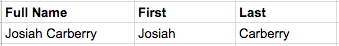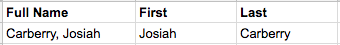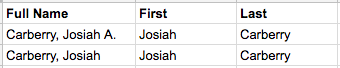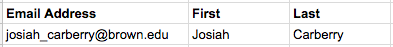# Extract Names from a Cell with an Excel Formula - Knowledgebase / Software / Microsoft Office - OIT Service Center# Extract Names from a Cell with an Excel Formula

Authors list

The following formulas will help you extract names out of cells in Excel (or Google Sheets) using a formula.

## First and Last Name

In our first example, the full name is in the format FirstName LastName.Assuming the full name is in cell A2, here are the formulas:

First Name:

`=LEFT(A2, FIND(" ", A2)-1)`

Last Name:

`=RIGHT(A2, FIND(" ", A2)+1)`

How it works:

This is a good demonstration of how to use the LEFT and RIGHT functions. LEFT gives you a specified number of characters (these could be letters, numbers, spaces, etc. - whatever is in the cell) from the left of a cell (in other words, the beginning of text in a cell), while RIGHT does the same from the right (end of the text in a cell). This is the "syntax" of the LEFT and RIGHT functions, or how they want you to give them the information they need:

=LEFT(which cell to look in, how many characters you want)

=RIGHT(which cell to look in, how many characters you want)

To find the first name, we start reading at the left of the cell, and stop right before the first space. In other words, the number of characters we want is one less than the position of the space. We use the FIND function to find the space, and then subtract 1 because we want to end at the character before the space.

To find the last name, we count from the right of the cell, and we want everything after the space. Similarly to the first name, we use FIND to find out where the space is, but this time we add 1 because our last name starts after the space.

## Last, First Name

In our second example, our full name is in the format Last, First. Again, we assume it's in cell A2.First name:

`=RIGHT(A5, LEN(A5)-FIND(", ", A5)-1)`

Last name:

`=LEFT(A2, FIND(", ", A2)-1)`

How it works:

This is very similar to the last set of formulas, except we are looking for a comma/space (", ") instead of just a space, and the last name is on the left, first name on the right.

## Last, First Name with Possible Middle Initial/Name

This third example is similar to the second, but it can handle names both with or without a middle initial/name. If your list of names might have middle names/initials, you might as well use this formula instead of the one above, but we included both so you can compare the formulas and better understand how they work.First Name:

`=if(LEN(A2)-LEN(SUBSTITUTE(A2," ",""))+1>2, MID(A2, FIND(", ", A2)+2, (FIND(" ", A2, FIND(", ", A2)+2)-FIND(", ", A2))-2), RIGHT(A2, LEN(A2)-FIND(", ", A2)-1))`

Last Name:

`=LEFT(A2, FIND(", ", A2)-1)`

This gets a little more complicated, so hang in there! If you haven't already, read the previous formula explanations to understand how the LEFT and RIGHT functions work.

This adds one additional text function - MID. Instead of counting from the beginning or end of a cell, the MID function can take text out of the middle. Here's the syntax, or format:

=MID(which cell to look in, which character to start at, how many characters you want)

We also use the FIND function, which finds the location of a character in the cell.

=FIND(what you're looking for, which cell to look in, [where to start looking])

The [where to start looking] is in brackets because it's optional.

There are two situations we might have - either we have just a first/last name, or a first/last/middle name. We determine which one is the case in this part of our formula:

LEN(A2)-LEN(SUBSTITUTE(A2," ",""))+1

If that is 2, we only have two names, and if it's greater than two, we have a middle name as well. This page explains how it works.

We use the IF function to test this and decide what to do in each situation. Here's the syntax:

=IF(thing to check, what to do if it's true, what to do if it's false)

In our case, we check if it's greater than 2...

if(LEN(A2)-LEN(SUBSTITUTE(A2," ",""))+1>2,

and if yes, we do this magic to find the first name between the two spaces in the phrase:

MID(A2, FIND(", ", A2)+2, (FIND(" ", A2, FIND(", ", A2)+2)-FIND(", ", A2))-2)

and if no, we use the simpler first name formula from the last example:

RIGHT(A2, LEN(A2)-FIND(", ", A2)-1)

Let's talk about the middle part of that function that uses the MID formula. In that part, we find the first name between the comma (", ") and second space in the cell. Here's an "english" translation of that part:

=MID(look in cell A2, start at two characters after the comma, take however many characters are between the first space and the second space)

How we start at two characters after the comma: FIND(", ", A2)+2 is two characters after the comma. We want two characters after, and not one as in previous examples, because there is both a comma and a space.

How we know how many characters are between the first and second space (which is the length of the name): (FIND(" ", A2, FIND(", ", A2)+2)-FIND(", ", A2))-2.

• To find the location of the second space: FIND(" ", A2, FIND(", ", A2)+2) We look for a space in A2, not starting at the beginning, but starting two characters after the comma
• Then, we subtract the start

The last name is very similar to the previous last name formula, but it looks for a comma (", ") instead of a space.

At Brown, most email addresses are in the format first_last@brown.edu. This formula helps extract first and last names from email addresses in this format.First Name:

`=PROPER(LEFT(A12, FIND("_", A2)-1))`

Last Name:

`=PROPER(MID(A12, FIND("_", A12)+1, FIND("@", A2)-FIND("_", A12)-1))`

### Contact the OIT Service Center

Phone: 401-863-4357

Email: help@brown.edu

### See our availability

https://it.brown.edu/get-help

### Walk-ins Welcome! Appointments recommended.

For reserved service for a technical consult or a loaner check-out, you can schedule an appointment here.

Report an Outage